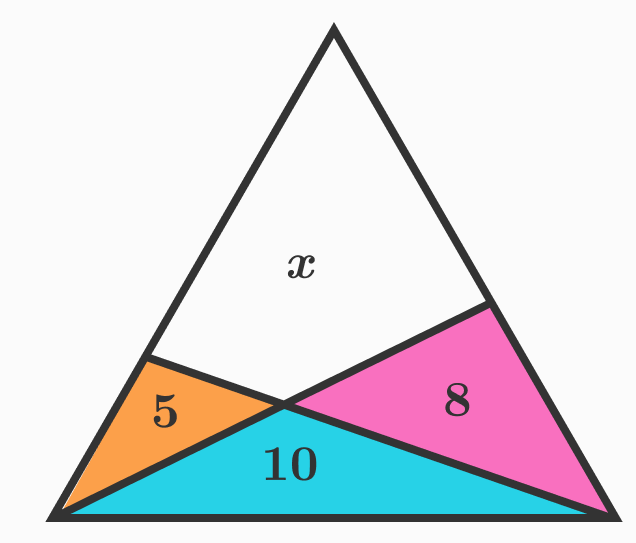# Area Properties Of Triangles

Geometry Level 3In the above triangle which is divided into four regions, the numbers each refer to the area of the corresponding region.

What is the value of $x$?

Note: Image not necessarily drawn up to scale.

×